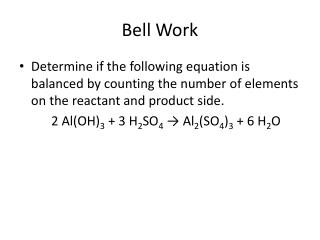Download PresentationBell Work

# Bell Work

Télécharger la présentation## Bell Work

- - - - - - - - - - - - - - - - - - - - - - - - - - - E N D - - - - - - - - - - - - - - - - - - - - - - - - - - -
##### Presentation Transcript

1. Bell Work • Determine if the following equation is balanced by counting the number of elements on the reactant and product side. 2 Al(OH)3 + 3 H2SO4 → Al2(SO4)3 + 6 H2O

2. Physical Science – Lecture 47 Balancing Equations

3. Law of Conservation of Mass • Law of Conservation of Mass says that in a chemical reaction, the mass of the products equals the mass of the reactants. • The number of each element (not compound) on each side must be equal since mass cannot be created or destroyed.

4. How do we count elements in equations? • Multiply the coefficient times the subscript for each element in a chemical formula. • If the chemical formula has parenthesis, the subscript outside the parenthesis is also multiplied by all elements inside the parenthesis.

5. Practice • CaCO3

6. Practice • Ca3(PO4)2

7. Practice • 3(NH4)3PO4

8. Practice • 4 FeI3

9. Practice • 2 Mg(NO3)2

10. Practice • 4 Be3(PO4)2

11. Balancing an Equation Rules • Apply the Law of Conservation of Mass to get the same number of atoms of every element on each side of the equation.

12. How to Balance an Equation • Step 1 - Draw a chart with elements from the reactant side on the left and elements from the product side on the right. (If one element appears in more than one formula, place the element in two separate blocks). • Step 2 – Identify the elements that are unbalanced. • Step 3 –Multiply each unbalanced element by any number that will make the two sides (reactant and product) equal. • Step 4 - When multiplying, if an element is in the same chemical formula as another, you must also multiply that element by the same number.

13. Why can’t we adjust the number of elements by changing the subscripts? • Balance chemical formulas by placing coefficients in front of them. Do not add subscripts, because this will change the chemical formulas.

14. Practice – Is it Balanced? • 3 Mg + N2→ Mg3N2

15. Practice – Is it balanced? • 3 Fe + 4 H2O → Fe3O4+ 4 H2

16. Practice- Is it balanced? • 4 H2O2→ 2 H2O + 3 O2

17. Practice – Is it Balanced? • 2 Fe + 3 O2→ 2 Fe2O3

18. Example – Balancing an Equation • Find the missing number to balance the equation: • 2 FeCl3 + 1 Be3(PO4)2 --> ___ BeCl2 + 2 FePO4

19. Practice – Balancing an Equation • Find the missing number to balance the equation: • ___ CH4+ 2 O2 --> 1 CO2 + 2 H2O

20. Practice – Balance the Equation • Find the missing number to balance the equation: • 2 Al + ___ HCl → 2 AlCl3+ 3 H2

21. Practice – Balance the Equation • Find the missing number to balance the equation: • 6 CO2+ 6 H2O → 1 C6H12O6+ ___ O2

22. Example – Balance the Following Equation • Al + O2 → Al2O3

23. Example – Balance the Following Equation • N2+ O2→ N2O

24. Example – Balance the Following Equation • Mg + O2 → MgO

25. Practice - Balance the Following Equation: • Al + CuO → Al2O3 + Cu

26. Practice - Balance the Following Equation: • HgO → Hg + O2

27. Practice - Balance the Following Equation: • K2O + H2O → KOH

28. Practice - Balance the Following Equation: • Mg + P4 → Mg3P2

29. Practice - Balance the Following Equation: • CaCl2 + AgNO3 → AgCl + Ca(NO3)2